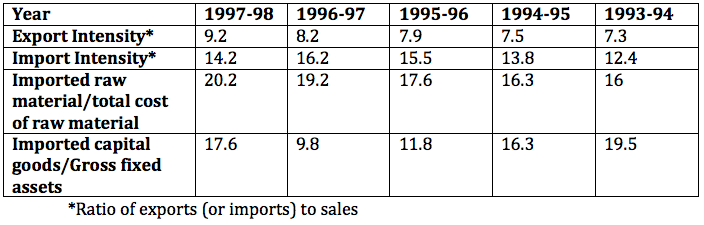### CAT 2000 Question Paper Question 23

Instructions

Directions for the next 4 questions: Answer these questions based on the table below:

The table shows trends in external transactions of Indian corporate sector during the period 1993-94 to 1997-98. In addition, following definitions hold good:

Sales, Imports, and Exports, respectively denote the sales, imports and exports in year i.

Deficit in year I, Deficit1 = Imports - Exports

Deficit Intensity in year I, DI = Deficit/Sales Growth rate of deficit intensity in year I, GDI = $$\frac{DI_i - DI_{i-1}}{DI_{i-1}}$$

Further, note that all imports are classified as either raw material or capital goods.

Trends in External Transactions of Indian Corporate Sector (All figures in %)Question 23

# The value of the highest growth rate in deficit intensity is approximately:

Solution

We know that  highest growth rate in deficit intensity was in the year 94-95 which is equal to 1.2*100/5.1 = 23.5% . hence option D.